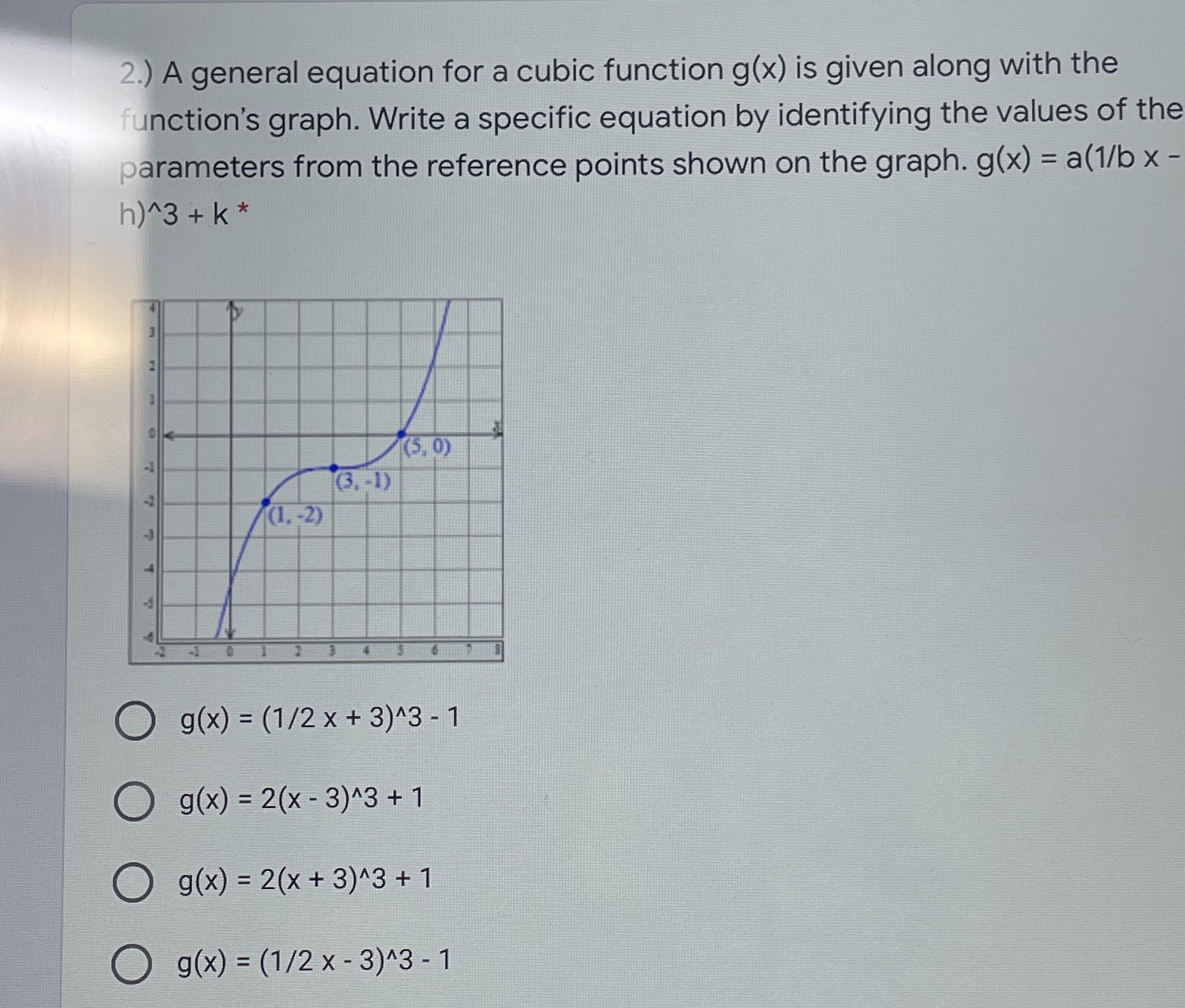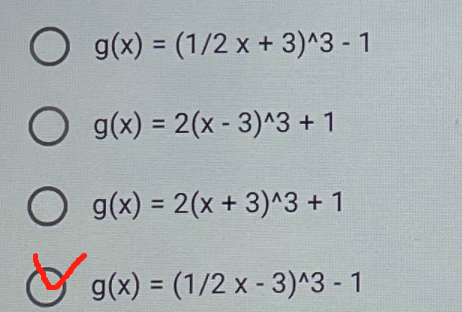### ¿Todavía tienes preguntas de matemáticas?

Pregunte a nuestros tutores expertos
Algebra
Pregunta2.) A general equation for a cubic function $$g ( x )$$ is given along with the function's graph. Write a specific equation by identifying the values of the parameters from the reference points shown on the graph. $$g ( x ) = a ( \frac{1}{b} x - h)^3+ k$$ .

A. $$g ( x ) = ( 1 / 2 x + 3 ) ^ { \wedge } 3 - 1$$

B. $$g ( x ) = 2 ( x - 3 ) ^ { \wedge } 3 + 1$$

C. $$g ( x ) = 2 ( x + 3 ) ^ { \wedge } 3 + 1$$

D. $$g ( x ) = ( 1 / 2 x - 3 ) ^ { \wedge } 3 - 1$$